# POJ 1330-Nearest Common Ancestors

POJ 1330-Nearest Common Ancestors
Nearest Common Ancestors
Time Limit: 1000MS Memory Limit: 10000K
Total Submissions: 19432 Accepted: 10292
Description
A rooted tree is a well-known data structure in computer science and engineering. An example is shown below: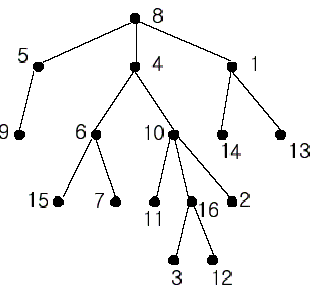In the figure, each node is labeled with an integer from {1, 2,...,16}. Node 8 is the root of the tree. Node x is an ancestor of node y if node x is in the path between the root and node y. For example, node 4 is an ancestor of node 16. Node 10 is also an ancestor of node 16. As a matter of fact, nodes 8, 4, 10, and 16 are the ancestors of node 16. Remember that a node is an ancestor of itself. Nodes 8, 4, 6, and 7 are the ancestors of node 7. A node x is called a common ancestor of two different nodes y and z if node x is an ancestor of node y and an ancestor of node z. Thus, nodes 8 and 4 are the common ancestors of nodes 16 and 7. A node x is called the nearest common ancestor of nodes y and z if x is a common ancestor of y and z and nearest to y and z among their common ancestors. Hence, the nearest common ancestor of nodes 16 and 7 is node 4. Node 4 is nearer to nodes 16 and 7 than node 8 is.
For other examples, the nearest common ancestor of nodes 2 and 3 is node 10, the nearest common ancestor of nodes 6 and 13 is node 8, and the nearest common ancestor of nodes 4 and 12 is node 4. In the last example, if y is an ancestor of z, then the nearest common ancestor of y and z is y.
Write a program that finds the nearest common ancestor of two distinct nodes in a tree.
Input
The input consists of T test cases. The number of test cases (T) is given in the first line of the input file. Each test case starts with a line containing an integer N , the number of nodes in a tree, 2<=N<=10,000. The nodes are labeled with integers 1, 2,..., N. Each of the next N -1 lines contains a pair of integers that represent an edge --the first integer is the parent node of the second integer. Note that a tree with N nodes has exactly N - 1 edges. The last line of each test case contains two distinct integers whose nearest common ancestor is to be computed.
Output
Print exactly one line for each test case. The line should contain the integer that is the nearest common ancestor.
Sample Input
2
16
1 14
8 5
10 16
5 9
4 6
8 4
4 10
1 13
6 15
10 11
6 7
10 2
16 3
8 1
16 12
16 7
5
2 3
3 4
3 1
1 5
3 5
Sample Output
4
3
Source
Taejon 2002

```#include<iostream>
#include<vector>
using namespace std;
int main()
{
//freopen("input.txt","r",stdin);
int t,n;
int node1,node2;
cin>>t;
while(t--)
{
cin>>n;
vector<int> s_f(n+1,0);//s_f[i]为第i个节点的父亲，开始时所有节点的父亲为0
for(int i=0;i<n-1;i++)
{
cin>>node1>>node2;
s_f[node2]=node1;//保存输入关系
}
cin>>node1>>node2;
vector<int> node1_father(n+1,0);//node1的父亲
node1_father[node1]=1;//自己是自己的祖先
while(s_f[node1]!=0)
{
node1_father[s_f[node1]]=1;//记录哪些点是node1的父亲
node1=s_f[node1];
}
if(node1_father[node2]!=0)//node2也是自己的祖先
{
cout<<node2<<endl;
continue;
}
while(s_f[node2]!=0)
{
if(node1_father[s_f[node2]]!=0)//从下网上找node2的祖先是否出现在node1的祖先里
{
cout<<s_f[node2]<<endl;
break;
}
else
node2=s_f[node2];
}
}
return 0;
}
```

# hihoCoder 1067-最近公共祖先·二

hihoCoder 1067-最近公共祖先·二
#1067 : 最近公共祖先·二

4
Adam Sam
Sam Joey
Sam Micheal
Adam Kevin
3
Sam Sam
Adam Sam
Micheal Kevin

Sam
Adam
Adam

hihoCoder确实是个练习算法的好网站，之前我们写过朴素的最近公共祖先问题：hihoCoder Problem 1062: 最近公共祖先·一，但那是一个效率比较低的方法，这一次，hihoCoder详细给我们介绍了最近公共祖先的离线算法，而且讲解非常详细。

```#include<iostream>
#include<string>
#include<map>
#include<vector>
using namespace std;
const int MAX_N=100010;//最大人数
map<string,int> name_index;//名字转换为数字
vector<string> index_name(MAX_N);//数字转换为名字
int color[MAX_N]={0};//0:白；1:灰；2:黑，初始颜色都是白色
int s_f[MAX_N];//son_father。s_f[i]表示第i个儿子的直接父亲
vector<vector<int> > f_s(MAX_N);//f_s[i]表示第i个父亲的所有孩子
vector<vector<int> > show_at(MAX_N);//show_at[i]表示第i个人在哪些询问里出现过
int q_l[MAX_N];//第i个询问的左边
int q_r[MAX_N];//第i个询问的右边
int father[MAX_N];//用于并查集，和s_f不一样
vector<string> ans;//最终结果
//保存某个人的信息，并返回其下标
int store_name(string name)
{
map<string,int>::iterator it=name_index.find(name);
if(it==name_index.end())//还不存在
{
int curr_index=name_index.size();//用当前已有人数作为其下标，正好是递增的。
name_index.insert(make_pair(name,curr_index));
index_name[curr_index]=name;//记录
father[curr_index]=curr_index;//初始的时候，并查集中每个元素都是一个独立的集合
return curr_index;//返回下标
}
else
return it->second;//已经存在，直接返回
}
//并查集FIND操作
int find_father(int name)
{
return name==father[name]?name:(father[name]=find_father(father[name]));
}
//并查集UNION操作
void union_father(int name1,int name2)
{
father[find_father(name1)]=find_father(name2);
}
//深度遍历族谱树
void DFS(int root)
{
color[root]=1;//首先修改颜色为灰色
int show_time=show_at[root].size();//查看该元素是否在询问里出现
if(show_time!=0)
{
for(int i=0;i<show_time;i++)//判断所有询问
{
int column=show_at[root][i];
int left=q_l[column];//找到该询问的左右元素
int right=q_r[column];
if(left==right)//如果是左右相同，则该自身即是其最近公共祖先
ans[column]=index_name[left];
else
{
if(left==root)//判断当前访问的是左边的还是右边的
{
if(color[right]==1)//另一个点为灰色
ans[column]=index_name[right];//结果即为该灰色点
else if(color[right]=2)//另一个点为黑色
ans[column]=index_name[find_father(right)];//找其向上最近的灰色节点
}
else
{
if(color[left]==1)//另一个点为灰色
ans[column]=index_name[left];
else if(color[left]=2)//另一个点为黑色
ans[column]=index_name[find_father(left)];
}
}
}
}
int sons=f_s[root].size();//查看是否有孩子节点
if(sons!=0)
{
for(int i=0;i<sons;i++)
DFS(f_s[root][i]);//深度遍历其所有孩子
}
color[root]=2;//改颜色为黑色
union_father(root,s_f[root]);//和直接父节点UNION
}
int main()
{
ios_base::sync_with_stdio(false);//取消同步，加速
cin.tie(0);
//freopen("input.txt","r",stdin);
int n,m;
string name1,name2;
int index1,index2;
cin>>n;
while(n--)
{
cin>>name1>>name2;
index1=store_name(name1);
index2=store_name(name2);
f_s[index1].push_back(index2);
s_f[index2]=index1;
}
cin>>m;
ans.resize(m);
for(int i=0;i<m;i++)
{
cin>>name1>>name2;
index1=store_name(name1);
index2=store_name(name2);
q_l[i]=index1;
q_r[i]=index2;
show_at[index1].push_back(i);
show_at[index2].push_back(i);
}
DFS(0);
for(int i=0;i<m;i++)
cout<<ans[i]<<endl;
return 0;
}
```

```vector<vector<int>> a; //sth
```

# POJ 1080-Human Gene Functions

POJ 1080-Human Gene Functions
Human Gene Functions
Time Limit: 1000MS Memory Limit: 10000K
Total Submissions: 17334 Accepted: 9637
Description
It is well known that a human gene can be considered as a sequence, consisting of four nucleotides, which are simply denoted by four letters, A, C, G, and T. Biologists have been interested in identifying human genes and determining their functions, because these can be used to diagnose human diseases and to design new drugs for them.
A human gene can be identified through a series of time-consuming biological experiments, often with the help of computer programs. Once a sequence of a gene is obtained, the next job is to determine its function.
One of the methods for biologists to use in determining the function of a new gene sequence that they have just identified is to search a database with the new gene as a query. The database to be searched stores many gene sequences and their functions – many researchers have been submitting their genes and functions to the database and the database is freely accessible through the Internet.
A database search will return a list of gene sequences from the database that are similar to the query gene.
Biologists assume that sequence similarity often implies functional similarity. So, the function of the new gene might be one of the functions that the genes from the list have. To exactly determine which one is the right one another series of biological experiments will be needed.
Your job is to make a program that compares two genes and determines their similarity as explained below. Your program may be used as a part of the database search if you can provide an efficient one.
Given two genes AGTGATG and GTTAG, how similar are they? One of the methods to measure the similarity
of two genes is called alignment. In an alignment, spaces are inserted, if necessary, in appropriate positions of
the genes to make them equally long and score the resulting genes according to a scoring matrix.
For example, one space is inserted into AGTGATG to result in AGTGAT-G, and three spaces are inserted into GTTAG to result in –GT--TAG. A space is denoted by a minus sign (-). The two genes are now of equal
length. These two strings are aligned:
AGTGAT-G
-GT--TAG
In this alignment, there are four matches, namely, G in the second position, T in the third, T in the sixth, and G in the eighth. Each pair of aligned characters is assigned a score according to the following scoring matrix.
![](http://poj.org/images/1080/1080_1.gif)
denotes that a space-space match is not allowed. The score of the alignment above is (-3)+5+5+(-2)+(-3)+5+(-3)+5=9.
Of course, many other alignments are possible. One is shown below (a different number of spaces are inserted into different positions):
AGTGATG
-GTTA-G
This alignment gives a score of (-3)+5+5+(-2)+5+(-1) +5=14. So, this one is better than the previous one. As a matter of fact, this one is optimal since no other alignment can have a higher score. So, it is said that the
similarity of the two genes is 14.
Input
The input consists of T test cases. The number of test cases ) (T is given in the first line of the input file. Each test case consists of two lines: each line contains an integer, the length of a gene, followed by a gene sequence. The length of each gene sequence is at least one and does not exceed 100.
Output
The output should print the similarity of each test case, one per line.
Sample Input
2
7 AGTGATG
5 GTTAG
7 AGCTATT
9 AGCTTTAAA
Sample Output
14
21
Source
Taejon 2001

1. s1取第i个基因，s2取'-'，则f[i,j]=f[i-1,j]+score[s1[i]],'-'];
2. s1取'-'，s2取第j个基因，则f[i,j]=f[i,j-1]+score['-',s2[j]];
3. s1取第i个基因，s2取第j个基因，则f[i,j]=f[i-1,j-1]+score[s1[i],s2[j]];

```#include<iostream>
#include<string>
using namespace std;
int score['T'+1]['T'+1];
int inf=-5;//负无穷
const int MAX_N=101;//基因最大长度
int f[MAX_N][MAX_N];//f[i][j]表示s1[0...i-1]和s2[0...j-1]的相识度
//初始化分数表
void init_score()
{
score['A']['A']=score['C']['C']=score['G']['G']=score['T']['T']=5;//可以连续赋值
score['-']['-']=inf;//负无穷
score['A']['C']=score['C']['A']=-1;
score['A']['G']=score['G']['A']=-2;
score['A']['T']=score['T']['A']=-1;
score['A']['-']=score['-']['A']=-3;//'-'的ASCII小于'T'
score['C']['G']=score['G']['C']=-3;
score['C']['T']=score['T']['C']=-2;
score['C']['-']=score['-']['C']=-4;
score['G']['T']=score['T']['G']=-2;
score['G']['-']=score['-']['G']=-2;
score['T']['-']=score['-']['T']=-1;
}
//动态规划求值
int DP(string s1,int n1,string s2,int n2)
{
f=0;
for(int i=1;i<=n1;i++)
//f[i]=f[i-1]+score['-'][s1[i-1]];//注意顺序不要弄错了，这里表示i,s1为纵轴；j,s2为横轴
f[i]=f[i-1]+score[s1[i-1]]['-'];
for(int j=1;j<=n2;j++)
//f[j]=f[j-1]+score[s2[j-1]]['-'];
f[j]=f[j-1]+score['-'][s2[j-1]];
for(int i=1;i<=n1;i++)
{
for(int j=1;j<=n2;j++)
{
int a=f[i-1][j]+score[s1[i-1]]['-'];
int b=f[i][j-1]+score['-'][s2[j-1]];
int c=f[i-1][j-1]+score[s1[i-1]][s2[j-1]];
int rs=a>b?a:b;
f[i][j]=rs>c?rs:c;//三者最大值
}
}
return f[n1][n2];
}
int main()
{
//freopen("input.txt","r",stdin);
int t;
cin>>t;
init_score();
while(t--)
{
int n1,n2;
string s1,s2;
cin>>n1>>s1>>n2>>s2;
cout<<DP(s1,n1,s2,n2)<<endl;
}
return 0;
}
```

# POJ 1094-Sorting It All Out

POJ 1094-Sorting It All Out
Sorting It All Out
Time Limit: 1000MS Memory Limit: 10000K
Total Submissions: 28397 Accepted: 9814
Description
An ascending sorted sequence of distinct values is one in which some form of a less-than operator is used to order the elements from smallest to largest. For example, the sorted sequence A, B, C, D implies that A < B, B < C and C < D. in this problem, we will give you a set of relations of the form A < B and ask you to determine whether a sorted order has been specified or not.
Input
Input consists of multiple problem instances. Each instance starts with a line containing two positive integers n and m. the first value indicated the number of objects to sort, where 2 <= n <= 26. The objects to be sorted will be the first n characters of the uppercase alphabet. The second value m indicates the number of relations of the form A < B which will be given in this problem instance. Next will be m lines, each containing one such relation consisting of three characters: an uppercase letter, the character "<" and a second uppercase letter. No letter will be outside the range of the first n letters of the alphabet. Values of n = m = 0 indicate end of input.
Output
For each problem instance, output consists of one line. This line should be one of the following three:
Sorted sequence determined after xxx relations: yyy...y.
Sorted sequence cannot be determined.
Inconsistency found after xxx relations.
where xxx is the number of relations processed at the time either a sorted sequence is determined or an inconsistency is found, whichever comes first, and yyy...y is the sorted, ascending sequence.
Sample Input
4 6
A<B
A<C
B<C
C<D
B<D
A<B
3 2
A<B
B<A
26 1
A<Z
0 0
Sample Output
Sorted sequence determined after 4 relations: ABCD.
Inconsistency found after 2 relations.
Sorted sequence cannot be determined.
Source
East Central North America 2001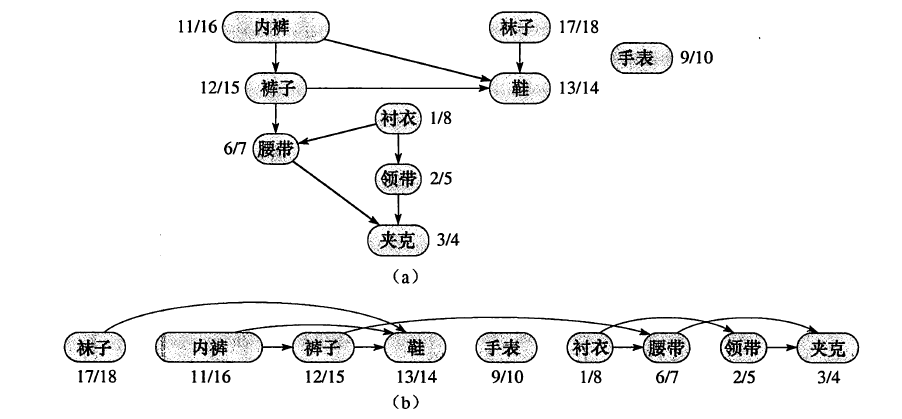Kahn算法

```L ← Empty list that will contain the sorted elements
S ← Set of all nodes with no incoming edges
while S is non-empty do
remove a node n from S
add n to tail of L
for each node m with an edge e from n to m do
remove edge e from the graph
if m has no other incoming edges then
insert m into S
if graph has edges then
return error (graph has at least one cycle)
else
return L (a topologically sorted order)
```

```L ← Empty list that will contain the sorted nodes
while there are unmarked nodes do
select an unmarked node n
visit(n)
function visit(node n)
if n has a temporary mark then stop (not a DAG)
if n is not marked (i.e. has not been visited yet) then
mark n temporarily
for each node m with an edge from n to m do
visit(m)
mark n permanently
unmark n temporarily
add n to head of L
```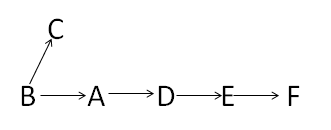```#include<iostream>
//#include<set>
#include<list>
#include<string>
#include<vector>
using namespace std;
int n,m;
const int MAX_N=26;
const int MAX_DIS=10000;
//*******这些都是维基百科关于拓扑排序（DFS版）里的变量含义
int temporary[MAX_N];
int permanent[MAX_N];
int marked[MAX_N];
//*******************************
int path[MAX_N][MAX_N];
//int dfs_visited[MAX_N];
list<int> L;//拓扑排序生产的顺序链
bool isDAG;//DAG=directed acyclic graph，无回路有向图
//每一个测试用例都要初始化路径
void init_path()
{
for(int i=0;i<n;i++)
for(int j=0;j<n;j++)
path[i][j]=0;
}
//每一次拓扑排序都要初始化temporary，permanent，marked
void init_tpm()
{
isDAG=true;
L.clear();
for(int i=0;i<n;i++)
{
temporary[i]=0;
permanent[i]=0;
marked[i]=0;
}
}
//递归访问。具体看维基百科
void visit(int i)
{
if(temporary[i]==1)
{
isDAG=false;
return;
}
else
{
if(marked[i]==0)
{
marked[i]=1;
temporary[i]=1;
for(int j=0;j<n;j++)
{
if(path[i][j]==1)
{
visit(j);
}
}
permanent[i]=1;
temporary[i]=0;
L.push_front(i);
}
}
}
/*
void init_dfs()
{
for(int i=0;i<n;i++)
dfs_visited[i]=0;
}*/
/*
//DFS有缺陷
void DFS(int v)
{
if(dfs_visited[v]==0)
{
dfs_visited[v]=1;
for(int i=0;i<n;i++)
{
if(dfs_visited[i]==0&&path[v][i]==1)
{
DFS(i);
}
}
}
}*/
//使用Floyd算法来判断生产的拓扑排序是否是严格有序的
bool Floyd()
{
int copy_path[MAX_N][MAX_N];
for(int i=0;i<n;i++)//首先复制一份路径图
for(int j=0;j<n;j++)
{
copy_path[i][j]=path[i][j];
if(i!=j&&copy_path[i][j]==0)//如果原来两点距离为0，说明他们是不直接连通的
copy_path[i][j]=MAX_DIS;//置为无穷
}
//floyd算法
for(int k=0;k<n;k++)
for(int i=0;i<n;i++)
for(int j=0;j<n;j++) if(copy_path[i][j]>copy_path[i][k]+copy_path[k][j])
copy_path[i][j]=copy_path[i][k]+copy_path[k][j];
vector<int> seq;//把原来用链表的拓扑序列转换成数组，方便后面的操作
list<int>::iterator it=L.begin();
while(it!=L.end())
{
seq.push_back(*it);
it++;
}
//如果这个拓扑链是严格有序的话，则前面的元素到后面的元素一定是可达的。
for(int i=0;i<n-1;i++)
{
for(int j=i+1;j<n;j++) { if(copy_path[seq[i]][seq[j]]>=MAX_DIS)//如果不可达，则不是严格有序的。
return false;
}
}
return true;
}
//拓扑排序DFS版本。返回0：有回路；1：虽然是拓扑排序，但非连通（不是严格有序）；2：是拓扑排序且连通（严格有序）（即任意两个元素都可以比较大小）
int topological_sorting()
{
for(int i=0;i<n;i++)
{
if(marked[i]==0)
{
visit(i);
}
}
if(!isDAG)
return 0;
else
{
/*init_dfs();
DFS(*L.begin());
for(int i=0;i<n;i++) { if(dfs_visited[i]==0) { return 1; } }*/ if(Floyd()) return 2; else return 1; } } int main() { //freopen("input.txt","r",stdin); string tmp; while(cin>>n>>m&&n&&m)
{
init_path();
vector<string> relations(m);
int i;
for(i=0;i<m;i++)//一次性把所有输入都存起来，免得后续麻烦 { cin>>relations[i];
}
int rs=-1;
for(i=0;i<m;i++)//每增加一个关系，都要重新拓扑排序一次
{
init_tpm();//每次都要初始化
tmp=relations[i];
path[tmp-'A'][tmp-'A']=1;
rs=topological_sorting();
if(rs==0)
{
cout<<"Inconsistency found after "<<i+1<<" relations."<<endl;
break;//如果是回路的话，后续的输入可以跳过
}
else if(rs==2)//成功
{
cout<<"Sorted sequence determined after "<<i+1<<" relations: ";
list<int>::iterator it=L.begin();
while(it!=L.end())
{
char c='A'+*it;
cout<<c;
it++;
}
cout<<"."<<endl;
break;//后续输入跳过
}
}
if(i==m&&rs==1)//如果处理完所有输入都没有形成严格有序的拓扑序列
cout<<"Sorted sequence cannot be determined."<<endl;
}
return 0;
}
```

# POJ 2263-Heavy Cargo

POJ 2263-Heavy Cargo
Heavy Cargo
Time Limit: 1000MS Memory Limit: 65536K
Total Submissions: 3549 Accepted: 1894
Description
Big Johnsson Trucks Inc. is a company specialized in manufacturing big trucks. Their latest model, the Godzilla V12, is so big that the amount of cargo you can transport with it is never limited by the truck itself. It is only limited by the weight restrictions that apply for the roads along the path you want to drive.
Given start and destination city, your job is to determine the maximum load of the Godzilla V12 so that there still exists a path between the two specified cities.
Input
The input will contain one or more test cases. The first line of each test case will contain two integers: the number of cities n (2<=n<=200) and the number of road segments r (1<=r<=19900) making up the street network.
Then r lines will follow, each one describing one road segment by naming the two cities connected by the segment and giving the weight limit for trucks that use this segment. Names are not longer than 30 characters and do not contain white-space characters. Weight limits are integers in the range 0 - 10000. Roads can always be travelled in both directions.
The last line of the test case contains two city names: start and destination.
Input will be terminated by two values of 0 for n and r.
Output
For each test case, print three lines:
a line saying "Scenario #x" where x is the number of the test case
a line saying "y tons" where y is the maximum possible load
a blank line
Sample Input
4 3
Karlsruhe Stuttgart 100
Stuttgart Ulm 80
Ulm Muenchen 120
Karlsruhe Muenchen
5 5
Karlsruhe Stuttgart 100
Stuttgart Ulm 80
Ulm Muenchen 120
Karlsruhe Hamburg 220
Hamburg Muenchen 170
Muenchen Karlsruhe
0 0
Sample Output
Scenario #1
80 tons
Scenario #2
170 tons
Source
Ulm Local 1998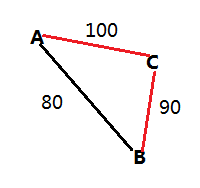```#include<iostream>
#include<map>
#include<string>
using namespace std;
const int MAX_N=202;
int load_ton[MAX_N][MAX_N];
//初始化数据
void init_load(int n)
{
for(int i=0;i<n;i++)
for(int j=0;j<n;j++)
load_ton[i][j]=0;
}
//改造Floyd算法
void Floyd(int n)
{
for(int k=0;k<n;k++)
{
for(int i=0;i<n;i++)
{
for(int j=0;j<n;j++)
{
int min_ton=load_ton[i][k]<load_ton[k][j]?load_ton[i][k]:load_ton[k][j];//这条路上的最小值即为能通过的最大值
if(load_ton[i][j]<min_ton)
{
load_ton[i][j]=min_ton;//对称矩阵
load_ton[j][i]=min_ton;//对称矩阵
}
}
}
}
}
int main()
{
//freopen("input.txt","r",stdin);
int n,r;
int case_num=1;
while(cin>>n>>r&&n&&r)
{
init_load(n);
map<string,int> city_index;//保存城市和下标的对应关系
string city1,city2;
int w;
int i=0;
while(r--)
{
cin>>city1>>city2>>w;
if(city_index.find(city1)==city_index.end())
city_index[city1]=i++;
if(city_index.find(city2)==city_index.end())
city_index[city2]=i++;
//load_ton[i-2][i-1]=w;//错，因为有可能cityi在之前已经加入了，这个时候i-1,i-2就不是cityi的下标了
//load_ton[i-1][i-2]=w;
load_ton[city_index[city1]][city_index[city2]]=w;//还是要从map里找对应下标
load_ton[city_index[city2]][city_index[city1]]=w;//因为路是两边通的，所以a[i][j]和a[j][i]相等
}
cin>>city1>>city2;
Floyd(n);
cout<<"Scenario #"<<case_num++<<endl;//
cout<<load_ton[city_index[city1]][city_index[city2]]<<" tons"<<endl<<endl;
}
return 0;
}
```

# POJ 2240-Arbitrage

POJ 2240-Arbitrage
Arbitrage
Time Limit: 1000MS Memory Limit: 65536K
Total Submissions: 16195 Accepted: 6814
Description
Arbitrage is the use of discrepancies in currency exchange rates to transform one unit of a currency into more than one unit of the same currency. For example, suppose that 1 US Dollar buys 0.5 British pound, 1 British pound buys 10.0 French francs, and 1 French franc buys 0.21 US dollar. Then, by converting currencies, a clever trader can start with 1 US dollar and buy 0.5 * 10.0 * 0.21 = 1.05 US dollars, making a profit of 5 percent.
Your job is to write a program that takes a list of currency exchange rates as input and then determines whether arbitrage is possible or not.
Input
The input will contain one or more test cases. Om the first line of each test case there is an integer n (1<=n<=30), representing the number of different currencies. The next n lines each contain the name of one currency. Within a name no spaces will appear. The next line contains one integer m, representing the length of the table to follow. The last m lines each contain the name ci of a source currency, a real number rij which represents the exchange rate from ci to cj and a name cj of the destination currency. Exchanges which do not appear in the table are impossible.
Test cases are separated from each other by a blank line. Input is terminated by a value of zero (0) for n.
Output
For each test case, print one line telling whether arbitrage is possible or not in the format "Case case: Yes" respectively "Case case: No".
Sample Input
3
USDollar
BritishPound
FrenchFranc
3
USDollar 0.5 BritishPound
BritishPound 10.0 FrenchFranc
FrenchFranc 0.21 USDollar
3
USDollar
BritishPound
FrenchFranc
6
USDollar 0.5 BritishPound
USDollar 4.9 FrenchFranc
BritishPound 10.0 FrenchFranc
BritishPound 1.99 USDollar
FrenchFranc 0.09 BritishPound
FrenchFranc 0.19 USDollar
Sample Output
Case 1: Yes
Case 2: No
Source
Ulm Local 1996

```#include<iostream>
#include<string>
#include<map>
using namespace std;
const int MAX_N=31;
double path[MAX_N][MAX_N];
//初始化，A到A的路径（汇率）为1；其他为0
void init_path(int n)
{
for(int i=0;i<n;i++)
{
for(int j=0;j<n;j++)
{
if(i==j)
path[i][j]=1.0;
else
path[i][j]=0.0;
}
}
}
//Floyd算法求所有点对的“最短距离”（最大乘积值）
void Floyd(int n)
{
for(int k=0;k<n;k++)
{
for(int i=0;i<n;i++)
{
for(int j=0;j<n;j++)
{
path[i][j]=(path[i][j]>path[i][k]*path[k][j])?path[i][j]:(path[i][k]*path[k][j]);
}
}
}
}
int main()
{
//freopen("input.txt","r",stdin);
int n,m;
int case_num=1;
while(cin>>n&&n)
{
init_path(n);
map<string,int> currency_index;//保存string和int的对应关系
int i=0;
string tmp;
for(int j=0;j<n;j++)
{
cin>>tmp;
currency_index[tmp]=i++;
}
cin>>m;
string ci,cj;
double rij;
while(m--)
{
cin>>ci>>rij>>cj;
path[currency_index[ci]][currency_index[cj]]=rij;
}
Floyd(n);
bool yes=false;
for(i=0;i<n;i++)
{
if(path[i][i]>1)
{
yes=true;
break;
}
}
if(yes)
cout<<"Case "<<case_num<<": Yes"<<endl;
else
cout<<"Case "<<case_num<<": No"<<endl;
case_num++;
}
return 0;
}
```

# POJ 3253-Fence Repair

POJ 3253-Fence Repair
Fence Repair
Time Limit: 2000MS Memory Limit: 65536K
Total Submissions: 27581 Accepted: 8976
Description
Farmer John wants to repair a small length of the fence around the pasture. He measures the fence and finds that he needs N (1 ≤ N ≤ 20,000) planks of wood, each having some integer length Li (1 ≤ Li ≤ 50,000) units. He then purchases a single long board just long enough to saw into the N planks (i.e., whose length is the sum of the lengths Li). FJ is ignoring the "kerf", the extra length lost to sawdust when a sawcut is made; you should ignore it, too.
FJ sadly realizes that he doesn't own a saw with which to cut the wood, so he mosies over to Farmer Don's Farm with this long board and politely asks if he may borrow a saw.
Farmer Don, a closet capitalist, doesn't lend FJ a saw but instead offers to charge Farmer John for each of the N-1 cuts in the plank. The charge to cut a piece of wood is exactly equal to its length. Cutting a plank of length 21 costs 21 cents.
Farmer Don then lets Farmer John decide the order and locations to cut the plank. Help Farmer John determine the minimum amount of money he can spend to create the N planks. FJ knows that he can cut the board in various different orders which will result in different charges since the resulting intermediate planks are of different lengths.
Input
Line 1: One integer N, the number of planks
Lines 2..N+1: Each line contains a single integer describing the length of a needed plank
Output
Line 1: One integer: the minimum amount of money he must spend to make N-1 cuts
Sample Input
3
8
5
8
Sample Output
34
Hint
He wants to cut a board of length 21 into pieces of lengths 8, 5, and 8.
The original board measures 8+5+8=21. The first cut will cost 21, and should be used to cut the board into pieces measuring 13 and 8. The second cut will cost 13, and should be used to cut the 13 into 8 and 5. This would cost 21+13=34. If the 21 was cut into 16 and 5 instead, the second cut would cost 16 for a total of 37 (which is more than 34).
Source
USACO 2006 November Gold

```#include<iostream>
#include<set>
using namespace std;
int main()
{
int n,tmp;
multiset<int> planks;
cin>>n;
while(n--)
{
cin>>tmp;
planks.insert(tmp);
}
//int rs=0;//超出最大值
long long rs=0;
int curr_sum;//当前两个元素之和
multiset<int>::iterator min_it;
while(planks.size()>1)
{
curr_sum=0;
min_it=planks.begin();//最小元素
rs+=*min_it;
curr_sum+=*min_it;
planks.erase(min_it);
min_it=planks.begin();//第二小元素
rs+=*min_it;
curr_sum+=*min_it;
planks.erase(min_it);
planks.insert(curr_sum);//插入新元素
}
cout<<rs;
return 0;
}
```

```#include<iostream>
#include<vector>
#include<list>
#include<algorithm>
using namespace std;
int main()
{
int n,tmp;
vector<int> planks;
cin>>n;
while(n--)
{
cin>>tmp;
planks.push_back(tmp);
}
sort(planks.begin(),planks.end());//首先排序
list<int> sorted_planks;
vector<int>::iterator it=planks.begin();
while(it!=planks.end())//把数组转换为链表，便于后面的插入删除操作
{
sorted_planks.push_back(*it);
it++;
}
long long rs=0;
int curr_sum;
list<int>::iterator min_it;
while(sorted_planks.size()>1)
{
curr_sum=0;
min_it=sorted_planks.begin();//最小元素
rs+=*min_it;
curr_sum+=*min_it;
sorted_planks.erase(min_it);
min_it=sorted_planks.begin();//第二小元素
rs+=*min_it;
curr_sum+=*min_it;
sorted_planks.erase(min_it);
min_it=sorted_planks.begin();
while(min_it!=sorted_planks.end()&&curr_sum>*min_it)//相当于插入排序
{
min_it++;
}
sorted_planks.insert(min_it,curr_sum);
}
cout<<rs;
return 0;
}
```

```min_it=sorted_planks.begin();
while(min_it!=sorted_planks.end()&&curr_sum>*min_it)//相当于插入排序
{
min_it++;
}
sorted_planks.insert(min_it,curr_sum);
```

list.insert(it,v)是将v插入到it的前面。需要特别注意的是while循环的条件，因为如果要将6插入到1,2,3,4里面，则如果任由min_it++的话，会超出链表的范围，所以还需要加上min_it!=sorted_planks.end()这个条件，但是把while写成这样：

```while(curr_sum>*min_it&&min_it!=sorted_planks.end())//相当于插入排序
{
min_it++;
}
```

# POJ 1068-Parencodings

POJ 1068-Parencodings
Parencodings
Time Limit: 1000MS Memory Limit: 10000K
Total Submissions: 20210 Accepted: 12196
Description
Let S = s1 s2...s2n be a well-formed string of parentheses. S can be encoded in two different ways:
q By an integer sequence P = p1 p2...pn where pi is the number of left parentheses before the ith right parenthesis in S (P-sequence).
q By an integer sequence W = w1 w2...wn where for each right parenthesis, say a in S, we associate an integer which is the number of right parentheses counting from the matched left parenthesis of a up to a. (W-sequence).
Following is an example of the above encodings:
S (((()()())))
P-sequence 4 5 6666
W-sequence 1 1 1456
Write a program to convert P-sequence of a well-formed string to the W-sequence of the same string.
Input
The first line of the input contains a single integer t (1 <= t <= 10), the number of test cases, followed by the input data for each test case. The first line of each test case is an integer n (1 <= n <= 20), and the second line is the P-sequence of a well-formed string. It contains n positive integers, separated with blanks, representing the P-sequence.
Output
The output file consists of exactly t lines corresponding to test cases. For each test case, the output line should contain n integers describing the W-sequence of the string corresponding to its given P-sequence.
Sample Input
2
6
4 5 6 6 6 6
9
4 6 6 6 6 8 9 9 9
Sample Output
1 1 1 4 5 6
1 1 2 4 5 1 1 3 9
Source
Tehran 2001

```#include<iostream>
#include<string>
#include<vector>
#include<stack>
using namespace std;
typedef struct PAR//括号结构
{
char c;//左括号或右括号
int pos;//该字符的下标
};
//根据P-sequence还原出括号字符串
string get_parentheses(vector<int>& p)
{
string rs="";
vector<int>::iterator it=p.begin();
int i=0;
while(it!=p.end())
{
while(i!=(*it))
{
rs+='(';
i++;
}
rs+=')';
it++;
}
return rs;
}
//根据括号字符串paren得到W-sequence
void get_w(vector<int>& w,string paren)
{
int len=paren.size();
stack<PAR> s_p;
PAR par;
vector<int> right_pos,left_pos;//分别记录某个右括号对应左括号的位置
for(int i=0;i<len;i++)
{
if(paren[i]=='(')//如果是左括号，入栈
{
par.c=paren[i];
par.pos=i;
s_p.push(par);
}
else//右括号，出栈，配对
{
par=s_p.top();
s_p.pop();
right_pos.push_back(i);
left_pos.push_back(par.pos);
}
}
vector<int>::iterator it_r=right_pos.begin();
vector<int>::iterator it_l=left_pos.begin();
while(it_r!=right_pos.end())//计算W-sequence
{
int num=0;
for(int i=*it_l;i<=*it_r;i++)
{
if(paren[i]==')')
num++;
}
w.push_back(num);
it_r++;
it_l++;
}
}
int main()
{
int t,n;
cin>>t;
while(t--)
{
cin>>n;
vector<int> p;//P-sequence
int tmp;
string parentheses;
while(n--)
{
cin>>tmp;
p.push_back(tmp);
}
parentheses=get_parentheses(p);
vector<int> w;//W-sequence
get_w(w,parentheses);
vector<int>::iterator it=w.begin();
while(it!=w.end())
{
cout<<*it<<" ";
it++;
}
cout<<endl;
}
return 0;
}
```

# hihoCoder 1066-无间道之并查集

hihoCoder 1066-无间道之并查集
#1066 : 无间道之并查集

10
0 Steven David
0 Lcch Dzx
1 Lcch Dzx
1 David Dzx
0 Lcch David
0 Frank Dzx
1 Steven Dzx
1 Frank David
0 Steven Dzx
0 Dzx Frank

yes
no
yes
yes

UNION操作就是已知元素c和d，要把这两个元素所在的集合合并起来，自然就是先用FIND找到c和d的祖先，然后把其中一个祖先当做另一个祖先的祖先，这样就把两个集合合并起来了。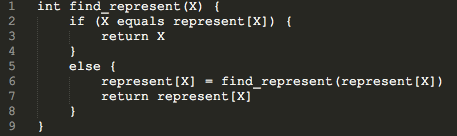```#include<iostream>
#include<string>
#include<map>
using namespace std;
map<string,string> represent;
//并查集FIND操作
string find_represent(string name)
{
if(name==represent[name])
return name;
else
{
represent[name]=find_represent(represent[name]);//把经过的节点全部链接到根节点
return represent[name];
}
}
int main()
{
//freopen("input.txt","r",stdin);
int n;
int op;
string name1,name2;
cin>>n;
while(n--)
{
cin>>op>>name1>>name2;
if(op==0)
{
if(represent.find(name1)==represent.end())
represent[name1]=name1;
if(represent.find(name2)==represent.end())
represent[name2]=name2;
represent[find_represent(name1)]=find_represent(name2);//UNION操作
}
else
{
//**********也需要先判断是否在map里*********
if(represent.find(name1)==represent.end())
represent[name1]=name1;
if(represent.find(name2)==represent.end())
represent[name2]=name2;
//******************************************
if(find_represent(name1)==find_represent(name2))
cout<<"yes"<<endl;
else
cout<<"no"<<endl;
}
}
return 0;
}
```

```if(name==represent[name])
```

# POJ 2965-The Pilots Brothers' refrigerator

POJ 2965-The Pilots Brothers' refrigerator
The Pilots Brothers' refrigerator
Time Limit: 1000MS Memory Limit: 65536K
Total Submissions: 19188 Accepted: 7358 Special Judge
Description
The game “The Pilots Brothers: following the stripy elephant” has a quest where a player needs to open a refrigerator.
There are 16 handles on the refrigerator door. Every handle can be in one of two states: open or closed. The refrigerator is open only when all handles are open. The handles are represented as a matrix 4х4. You can change the state of a handle in any location [i, j] (1 ≤ i, j ≤ 4). However, this also changes states of all handles in row i and all handles in column j.
The task is to determine the minimum number of handle switching necessary to open the refrigerator.
Input
The input contains four lines. Each of the four lines contains four characters describing the initial state of appropriate handles. A symbol “+” means that the handle is in closed state, whereas the symbol “−” means “open”. At least one of the handles is initially closed.
Output
The first line of the input contains N – the minimum number of switching. The rest N lines describe switching sequence. Each of the lines contains a row number and a column number of the matrix separated by one or more spaces. If there are several solutions, you may give any one of them.
Sample Input
-+--
----
----
-+--
Sample Output
6
1 1
1 3
1 4
4 1
4 3
4 4
Source
Northeastern Europe 2004, Western Subregion

```-+--
----
----
-+--
```

```typedef struct P//翻转时的格子坐标
{
int x,y;
};
P trace[MAX_STATUS];//trace[i]到达i状态值时翻转的某个格子坐标
int pre[MAX_STATUS];//pre[i]记录i状态的前一个状态值
```

pre[i]数组用来记录到达i状态时的前一个状态，而trace[i]则用来记录到达该状态时翻转的格子坐标。这样通过成功状态回溯到开始状态就可以找到所有路径了，具体实现请看下面的代码。

```#include<iostream>
#include<queue>
#include<vector>
using namespace std;
const int MAX_STATUS=65536;//2^16=65536种，所以[0,65535]
const int ALL_1=65535;//全1
int visited[MAX_STATUS]={0};//访问标记
int XOR={0x111,0x1011,0x1101,0x1110};//列异或值
typedef struct POS//position翻转位置
{
int val;//当前翻转状态的值
int step;//到当前状态共翻转了多少次
};
typedef struct P//翻转时的格子坐标
{
int x,y;
};
P trace[MAX_STATUS];//trace[i]到达i状态值时翻转的某个格子坐标
int pre[MAX_STATUS];//pre[i]记录i状态的前一个状态值
//通过反向索引打印结果
void print_result(POS pos,int init_id)
{
cout<<pos.step<<endl;
vector<P> points;
points.push_back(trace[pos.val]);
int pre_val=pre[pos.val];
while(pre_val!=init_id)//当没有追溯到初始状态时，一直循环
{
points.push_back(trace[pre_val]);
pre_val=pre[pre_val];//追溯
}
int p_num=points.size();//顺着打印出来
for(int i=p_num-1;i>=0;i--)
cout<<points[i].x<<" "<<points[i].y<<endl;
}
//广度优先搜索
void BFS(int init_id)
{
POS p,next_p;
p.val=init_id;
p.step=0;
visited[p.val]=1;
pre[p.val]=-1;//没有前一个状态
queue<POS> Q_P;
Q_P.push(p);
while(!Q_P.empty())
{
p=Q_P.front();
Q_P.pop();
if(p.val==ALL_1)
{
print_result(p,init_id);
return;
}
for(int i=0;i<4;i++)
{
int curr_xor=XOR[i];//i不同时，翻转异或的值也不同
for(int j=0;j<4;j++)
{
next_p.val=p.val;
next_p.val^=0xf<<(4*(3-i));//横向翻转
next_p.val^=curr_xor<<(3-j);//纵向翻转
if(visited[next_p.val]==0)
{
visited[next_p.val]=1;
next_p.step=p.step+1;
pre[next_p.val]=p.val;//记录前一个状态值
trace[next_p.val].x=i+1;//注意输出的坐标是从1开始到4
trace[next_p.val].y=j+1;//所以这里加1
Q_P.push(next_p);
}
}
}
}
}
int main()
{
//freopen("input.txt","r",stdin);
char c;
int id=0;
for(int i=0;i<4;i++)
{
for(int j=0;j<4;j++)
{
cin>>c;
id<<=1;//id=id<<1;
if(c=='-')//-:1;+:0
id+=1;
}
}
BFS(id);
return 0;
}
```